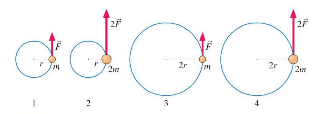# Problem: Balls are attached to light rods and can move in horizontal circles as shown in the figure. Rank in order, from smallest to largest, the torques T1 to T4 about the centers of the circles. Explain.

###### FREE Expert Solution

Torque:

$\overline{){\mathbit{\tau }}{\mathbf{=}}{\mathbit{r}}{\mathbit{F}}}$

We do not need the mass to calculate torque.

94% (178 ratings)###### Problem Details

Balls are attached to light rods and can move in horizontal circles as shown in the figure. Rank in order, from smallest to largest, the torques T1 to T4 about the centers of the circles. Explain.